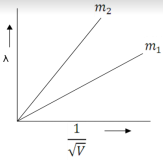# NEET Questions Solved

BOARD

Plot a graph showing variation of de-Brogile wavelength $\lambda$. versus $\frac{1}{\sqrt{V}}$,where V is accelerating potential for two particles A and B carrying same charge but of masses ${m}_{1}{m}_{2}\left({m}_{1}>{m}_{2}\right)$. Which one of the two represents a particle of smaller mass and why?               (2)$\left(1}{2}\right)$

As $\lambda =\frac{h}{\sqrt{2mqv}}$

As the  charge of two particles is same,therfore         $\left(1}{2}\right)$

$\frac{\lambda }{\left(\frac{1}{\sqrt{v}}\right)}\alpha \frac{1}{\sqrt{m}}$ i.e.    slope$\alpha \frac{1}{\sqrt{m}}$

Hence,particle with lower mass$\left({m}_{2}\right)$will have greater slope.$\left(1}{2}\right)$

Difficulty Level:

Crack NEET with Online Course - Free Trial (Offer Valid Till August 23, 2019)